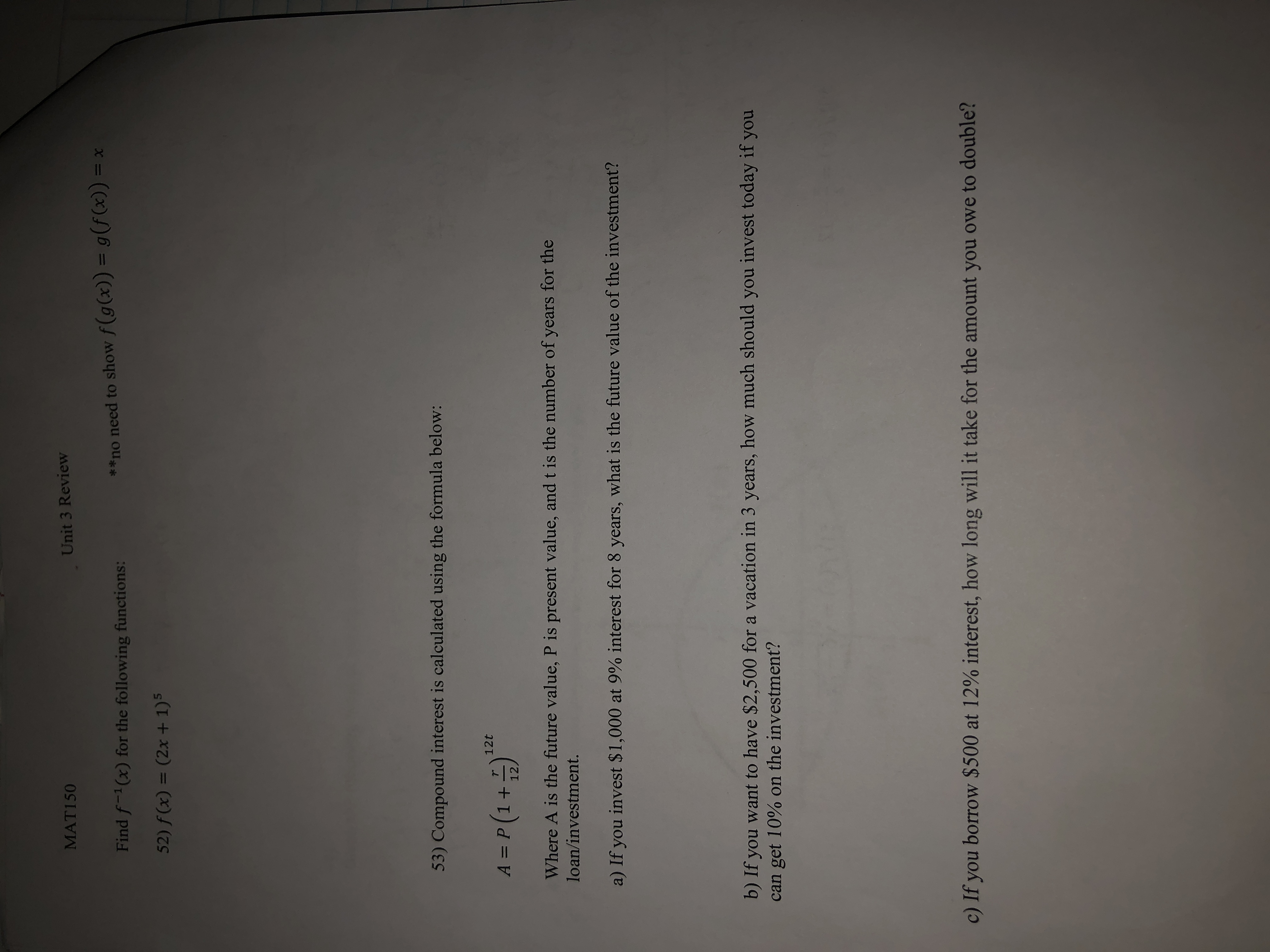# MAT150Unit 3 Review**no need to show f(g(x)) = g(f(x)) = xFind f(x) for the following functions:52) f(x) = (2x +1)553) Compound interest is cal culated using the formula below:A = P (1+12Where A is the future value, P is present value, and t is the number of years for theloan/investment.a) If you invest \$1,000 at 9% interest for 8 years, what is the future value of the investment?b) If you want to have \$2,500 for a vacation in 3 years, how much should you invest today if youcan get 10% on the investment?c) If you borrow \$500 at 12% interest, how long will it take for the amount you owe to double?

Questionhelp_outlineImage TranscriptioncloseMAT150 Unit 3 Review **no need to show f(g(x)) = g(f(x)) = x Find f(x) for the following functions: 52) f(x) = (2x +1)5 53) Compound interest is cal culated using the formula below: A = P (1+12 Where A is the future value, P is present value, and t is the number of years for the loan/investment. a) If you invest \$1,000 at 9% interest for 8 years, what is the future value of the investment? b) If you want to have \$2,500 for a vacation in 3 years, how much should you invest today if you can get 10% on the investment? c) If you borrow \$500 at 12% interest, how long will it take for the amount you owe to double? fullscreen
check_circleExpert Solution
Step 1

“Since you have asked multiple question, we will solve the first question for you. If you want any specific question to be solved then please ...

### Want to see the full answer?

See Solution

#### Want to see this answer and more?

Solutions are written by subject experts who are available 24/7. Questions are typically answered within 1 hour*

See Solution
*Response times may vary by subject and question
Tagged in

### Other